# Principal focal point and refractive index (n)

Unlike mirrors, different convex lenses of the same size (i.e. ones with the same centre of curvature which defines how curved the convex lens is) can have different principal focal points.

So much depends on the material the convex lens is made of and the angle of refraction that this creates. You have to know the refraction index of the material (n).

Example 1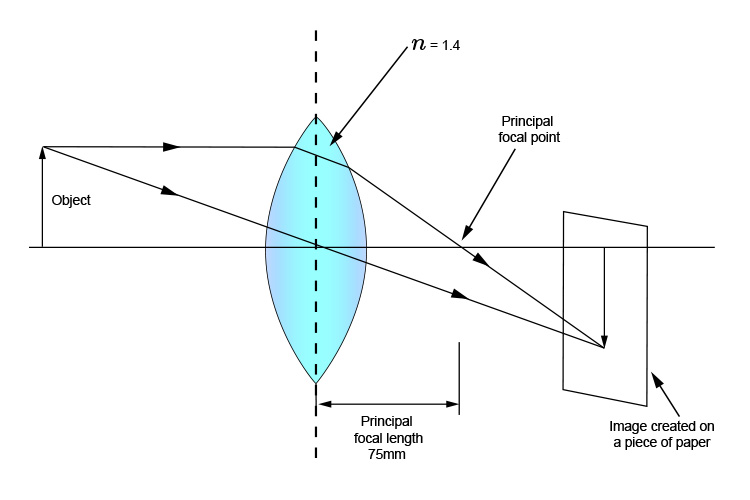Example 2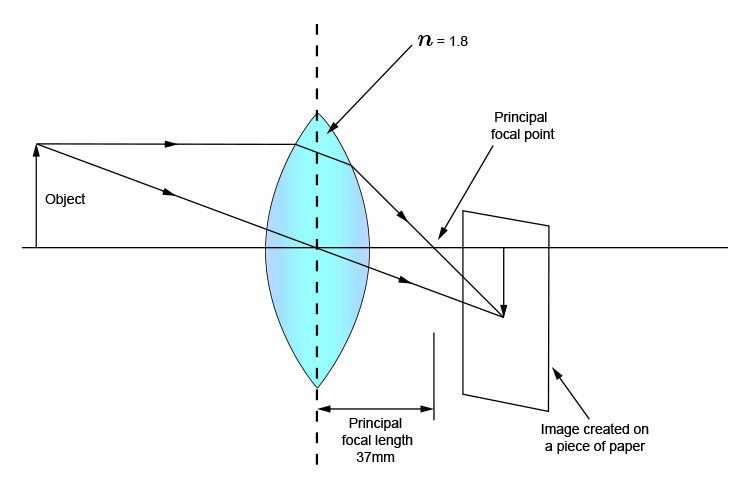NOTE: For comparison, Air n=1,    Glass n=1.5,    Diamond n=2.4This makes sense and should be easy to remember because the higher the refractive index the more the light ray refracts (see refractive index section).

Below are the figures used to work this out but they should not concern anyone at this level.

Example 1

 Lens radius = 60mm Index of refraction n= 1.4 Object position = 135mm\ \ \ (d\o) Object height = 60mm Gives Image position = 168mm\ \ \ (d\i) Image height = 74mm Focal length = 75mm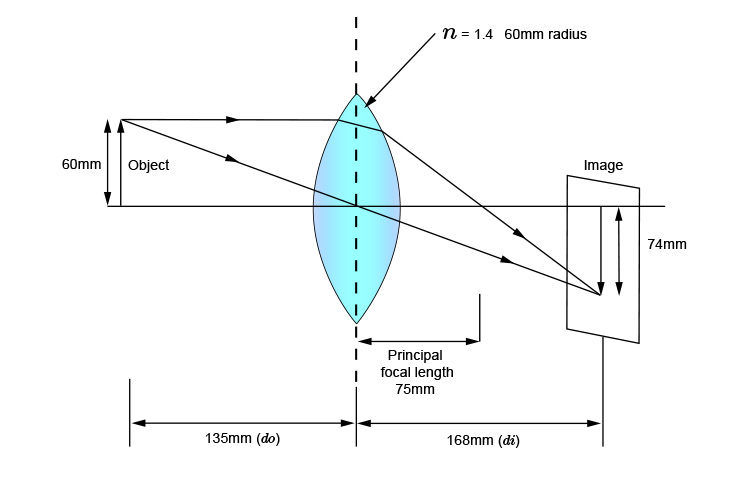Example 2

 Lens radius = 60mm Index of refraction n= 1.8 Object position = 135mm\ \ \ (d\o) Object height = 60mm Gives Image position = 51mm\ \ \ (d\i) Image height = 22mm Focal length = 37mm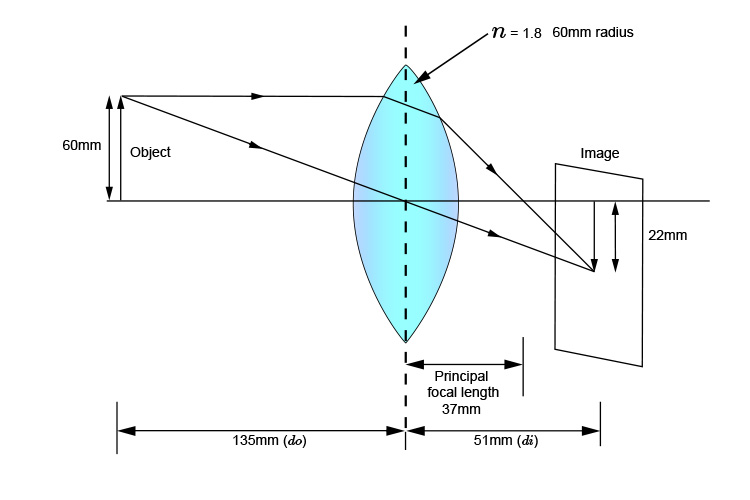NOTE:

In some books you may read that the principal focal length of a lens is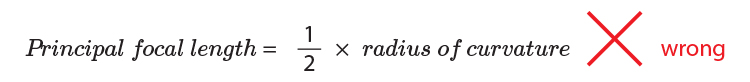You can see from the above that this can not possibly be correct because for the same curvature lens different materials will have a different principal focal point, hence a different principal focal length.